﻿ 国内国际双循环：理论框架与中国实践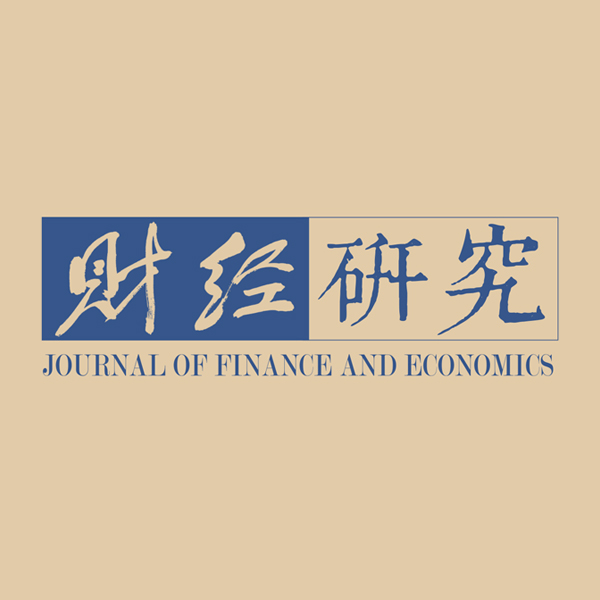《财经研究》
2021第47卷第4期

Dual Circulations of Domestic and International Economy: Theoretical Framework and Chinese Practice
Li Feng
Institute of World Economy，Jiangsu Academy of Social Sciences，Nanjing 210004，China
Summary: Facing the current anti-globalization trend of rising protectionism, sluggish international trade and investment, as well as the impact of the new pandemic on the global industrial chain and supply chain, the CPC Central Committee duly put forward the strategic plan of “accelerating the formation of a new development pattern featuring domestic circulation as the main body and mutually reinforcing the dual circulations of domestic and international economy”. What is the connotation of domestic circulation, and how to measure it? What is the development trend of the dual circulations of domestic and international economy? How do the dual circulations of domestic and international economy affect each other, and what are the problems? How should we further build a new development pattern? All these problems are worth considering. This paper clarifies the connotation and boundary of domestic circulation and international circulation from the theoretical level, constructs the measurement method of dual circulations by using the input-output model, and thus constructs the theoretical analysis framework of dual circulations. In this way, we can study the current situation, characteristics, interaction and mechanism of China’s dual circulations.It comes to the following conclusions: Firstly, based on domestic supporting capacity and domestic market space, China’s economic development has shown a significant feature of domestic circulation and the overall level is higher than that of most emerging economies. Secondly, the general trade export relying on the domestic supply system is the main mode for China to participate in international circulation, followed by the processing trade mode of “both ends outside” and the general trade import mode using foreign supporting facilities. Thirdly, the entry of foreign-owned enterprises, especially cost-oriented foreign-owned enterprises greatly hinders the construction of domestic market network. Fourthly, the enhancement of domestic circulation, especially domestic supporting capacity, has significantly promoted general trade exports with a higher value-added level, which is conducive to the transformation and upgrading of China’s open economy.The conclusions of this paper provide important enlightenment for China to accelerate the construction of a new development pattern. In short, this paper mainly expands the existing research from the following aspects: Firstly, the input-output method is used to construct the theoretical analysis framework of dual circulations, which provides a normative analysis method for the study of dual circulations. Secondly, using the data of national input-output to make a quantitative analysis of the development situation and characteristics of dual circulations of major countries, especially China, is helpful to deeply analyze the current situation and prominent problems of China’s dual circulations. Thirdly, the policy suggestions based on normative research and analysis conclusions have certain practical significance for accelerating the construction of new development pattern.
Key words: domestic circulation    international circulation    intensity of domestic circulation    intensity of international circulation

20世纪90年代中期以来，通过承接发达国家产业梯度转移融入全球价值链分工，以及充分利用跨国公司全球供应链和市场营销网络，中国生产制造及出口能力迅速提升，发展成为开放型经济大国。以经济开发区、出口加工区、保税区、高新技术开发区等各种“经济飞地”为载体，以外资为主导，以代工制造、加工贸易为依托的出口导向型经济模式成为中国开放型经济发展的主要经验。然而，该发展模式不可避免地存在着一些瓶颈和制约，具体而言：一是随着国内生产要素成本的不断上升，“全球制造基地”的传统发展路径难以持续，而迈向“微笑曲线”两端的价值链攀升之路又遭到发达国家的百般阻扰；二是在对出口市场及进口中间品高度依赖的同时，对国内上下游资源要素的整合力度相对不够；三是以外资企业为主导的出口导向型模式在某种程度上影响了内资企业成长及国内市场培育；四是各地区在推进对外开放过程中呈现出一定程度的“各自为政”，不利于国内资源统筹基础上的区域一体化进程。

（一）国内国际双循环的内涵及边界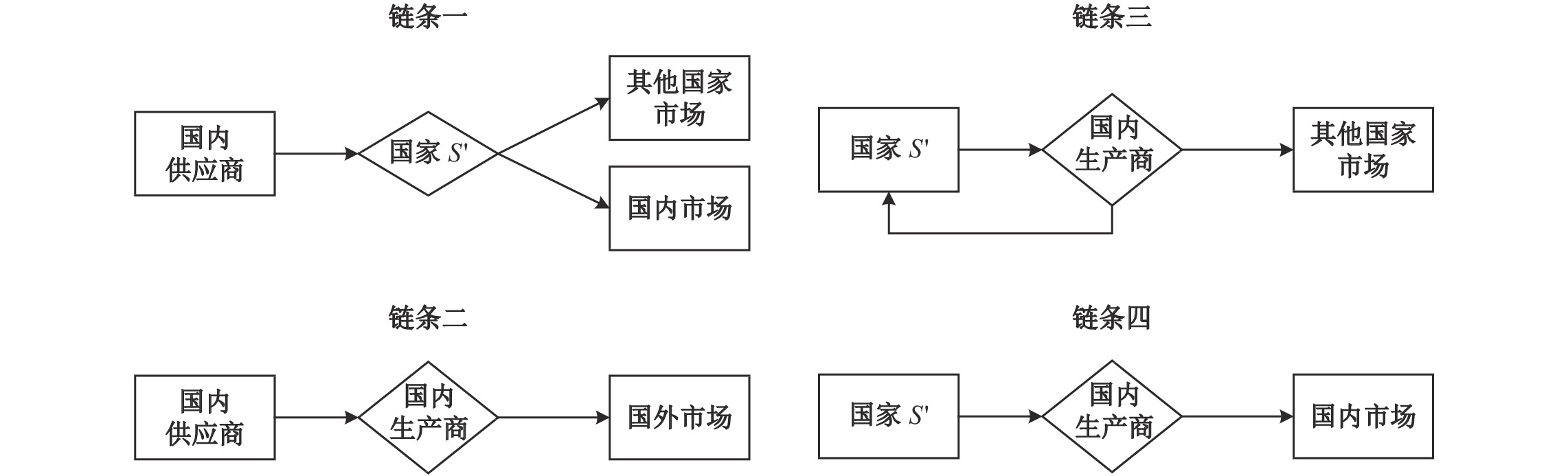图 1 国际循环的各种链条形式

（二）国内国际双循环的辩证关系

（三）国内国际双循环的度量方法

 ${X^R}{\rm{ = }}\sum\limits_S {{X^{RS}}} {\rm{ + }}{X^{RR}}$ (1)

${X^R}$

$\sum\limits_S {{X^{RS}}}$
${X^{RR}}$

${X^{RS}}$

${X^{RS}}{\rm{ = }}{A^{RS}}{X^S}{\rm{ + }}{Y^{RS}}$
${A^{RS}}$

${X^S}$

${Y^{RS}}$

 \begin{aligned} {X^{RS}}{\rm{ = }}& U({A^{RS}}{X^{\rm{S}}}{\rm{ + }}{Y^{RS}}) = ({V^R}{B^{RR}}{\rm{ + }}{V^S}{B^{SR}}{\rm{ + }}\sum\limits_{{{T}} \ne {{S,R}}} {{V^T}{B^{TR}}} )({Y^{RS}}{\rm{ + }}{A^{RS}}{X^S}) \\ =& {V^R}{B^{RR}}{Y^{RS}}{\rm{ + }}{V^S}{B^{SR}}{Y^{RS}}{\rm{ + }}\sum\limits_{{{T}} \ne {{S,R}}} {{V^T}{B^{TR}}} {Y^{RS}}{\rm{ + }}{V^R}{B^{RR}}{A^{RS}}{X^S}{\rm{ + }}{V^S}{B^{SR}}{A^{RS}}{X^S}{\rm{ + }}\sum\limits_{{{T}} \ne {{S,R}}} {{V^T}{B^{TR}}} {A^{RS}}{X^S} \end{aligned} (2)

${X^S}{\rm{ = }}{L^{SS}}{Y^S}$
${Y^S}$

 ${X^{RS}}{\rm{ = }}{}_{\rm{1}}{V^R}{B^{RR}}{Y^{RS}}{\rm{ + }}{}_{\rm{2}}{V^S}{B^{SR}}{Y^{RS}}{\rm{ + }}{}\sum\limits_{{_3{T}} \ne {{S,R}}} {{V^T}{B^{TR}}} {Y^{RS}}{\rm{ + }}{}_{\rm{4}}{V^R}{B^{RR}}{A^{RS}}{L^{SS}}{Y^S}{\rm{ + }}{}_{\rm{5}}{V^S}{B^{SR}}{A^{RS}}{L^{SS}}{Y^S}{\rm{ + }}{}\sum\limits_{_6{{T}} \ne {{S,R}}} {{V^T}{B^{TR}}} {A^{RS}}{L^{SS}}{Y^S}$ (3)

${X^{RR}}$

 \begin{aligned} {X^{RR}}{\rm{ = }} & U{\rm{(}}{A^{RR}}{X^R}{\rm{ + }}{Y^{RR}}{\rm{) = }}({V^R}{B^{RR}} + {V^S}{B^{SR}} + \sum\limits_{T \ne S,R} {{V^T}{B^{TR}}} )({A^{RR}}{X^R} + {Y^{RR}}) \\ {\rm{ = }} & {}_{\rm{7}}{V^R}{B^{RR}}{Y^{RR}}{\rm{ + }}{}_{\rm{8}}{V^R}{B^{RR}}{A^{RR}}{L^{RR}}{Y^{RR}}{\rm{ + }}{}_{\rm{9}}{V^R}{B^{RR}}{A^{RR}}{L^{RR}}{Y^{RW}}{\rm{ + }}{}_{{\rm{10}}}{V^S}{B^{SR}}{Y^{RR}} \\ & + _{{\rm{11}}}{V^S}{B^{SR}}{A^{RR}}{L^{RR}}{Y^R}{\rm{ + }}{}\sum\limits_{_{12}{{T}} \ne {{S,R}}} {{V^T}{B^{TR}}} {Y^{RR}}{\rm{ + }}\sum\limits_{_{13}{{T}} \ne {{S,R}}} {{V^T}{B^{TR}}} {A^{RR}}{L^{RR}}{Y^R} \end{aligned} (4)

${Y^R}$

${Y^{RR}}$

${Y^{RW}}$

 \begin{aligned} {X^R}{\rm{ = }} & X_{DC}^R{\rm{ + }}X_{IC}^R{\rm{ = }}X_{DC}^R{\rm{ + (}}X_{IC1}^R + X_{IC2}^R{\rm{ + }}X_{IC3}^R{\rm{)}} \\ {\rm{ = }} & ({}_{\rm{7}}{V^R}{B^{RR}}{Y^{RR}}{\rm{ + }}{}_{\rm{8}}{V^R}{B^{RR}}{A^{RR}}{L^{RR}}{Y^{RR}}{{\rm{)}}_{DC}} + {{\rm{(}}\sum\limits_{_4S}{{V^R}{B^{RR}}{A^{RS}}{L^{SS}}{Y^S}} {\rm{ + }}\sum\limits_{_1S} {{V^R}{B^{RR}}{Y^{RS}}} {\rm{ + }}{}_{\rm{9}}{V^R}{B^{RR}}{A^{RR}}{L^{RR}}{Y^{RW}}{\rm{)}}_{IC1}} \\ &{\rm{ + (}}{}\sum\limits_{_2S} {{V^S}{B^{SR}}{Y^{RS}}} {\rm{ + }}{}\sum\limits_{_3{{T}} \ne {{S,R}}} {\sum\limits_{S} {{V^T}{B^{TR}}} {Y^{RS}}} {\rm{ + }}{}\sum\limits_{_5S} {{V^S}{B^{SR}}{A^{RS}}{L^{SS}}{Y^S}} {\rm{ + }}\sum\limits_{_6{{T}} \ne {{S,R}}} {\sum\limits_S {{V^T}{B^{TR}}} {A^{RS}}{L^{SS}}{Y^S}} {{\rm{)}}_{IC2}} \\ &{\rm{ + (}}{}_{{\rm{10}}}{V^S}{B^{SR}}{Y^{RR}}{\rm{ + }}{}_{{\rm{11}}}{V^S}{B^{SR}}{A^{RR}}{L^{RR}}{Y^R}{\rm{ + }}{}\sum\limits_{_{12}{{T}} \ne {{S,R}}} {{V^T}{B^{TR}}} {Y^{RR}}{\rm{ + }}{}\sum\limits_{_{13}{{T}} \ne {{S,R}}} {{V^T}{B^{TR}}} {A^{RR}}{L^{RR}}{Y^R}{{\rm{)}}_{IC3}} \\ \end{aligned} (5)

$X_{DC}^R$
$X_{IC}^R$

$X_{IC}^R$

$X_{IC1}^R$

$X_{IC2}^R$
（对应国际循环链条三）情况，而第10–13项（对应国际循环链条四）是“进口配套+国内市场”的一般贸易进口
$X_{IC3}^R$

 $ID{C^R}{\rm{ = }}{{X_{DC}^R} / {{X^R}}}$ (6)

$ID{C^R}$

 $ID{C^R}{\rm{ = }}{{{V^R}{B^{RR}}{Y^{RR}}} / {{X^R}}}{\rm{ + }}{{{V^R}{B^{RR}}{A^{RR}}{L^{RR}}{Y^{RR}}} / {{X^R}}}{\rm{ = }}{{({V^R}{B^{RR}}{\rm{ + }}{V^R}{B^{RR}}{A^{RR}}{L^{RR}}) \times {Y^{RR}}} / {{X^R}}}$ (7)

${V^R}{B^{RR}}{\rm{ + }}{V^R}{B^{RR}}{A^{RR}}{L^{RR}}$
）表示产品生产过程的国内关联程度，度量的是国内配套关联水平（Intensity of domestic supporting correlationIDS）；而
${{{Y^{RR}}} / {{X^R}}}$

 $II{C^R}{\rm{ = }}{{X_{IC}^R} / {{X^R}}}$ (8)

 $II{C^R}{\rm{ = }}IIC_1^R + IIC_2^R + IIC_3^R{\rm{ = }}{{X_{IC1}^R} / {{X^R}}} + {{X_{IC2}^R} / {{X^R}}} + {{X_{IC3}^R} / {{X^R}}}$ (9)

$IIC_1^R$

$IIC_2^R$

$IIC_3^R$

（一）中国国内循环的发展态势及特征

1. 国家层面的核算。基于国内循环强度统计指标，本文可以利用WIOD数据库对中国国内循环的发展态势进行分析。首先，在核算行业层面国内循环强度的基础上，以各制造部门的产出占比为权重加总核算出中国制造部门的国内循环强度的整体水平。图2显示了2000年以来中国制造部门的国内循环强度发展态势，可见2001年“入世”显著地推进了中国贸易自由化进程，进出口关税削减大幅降低了中国融入国际循环的成本（毛其淋和盛斌，2013），导致中国制造部门的国内循环强度在“入世”之初逐年降低并达到2004年0.542的低点。在此之后，中国制造部门的国内循环强度迅速上扬并于2014年达到0.655的水平，表明整体而言中国实际上已经是一个以国内循环为主体的国家。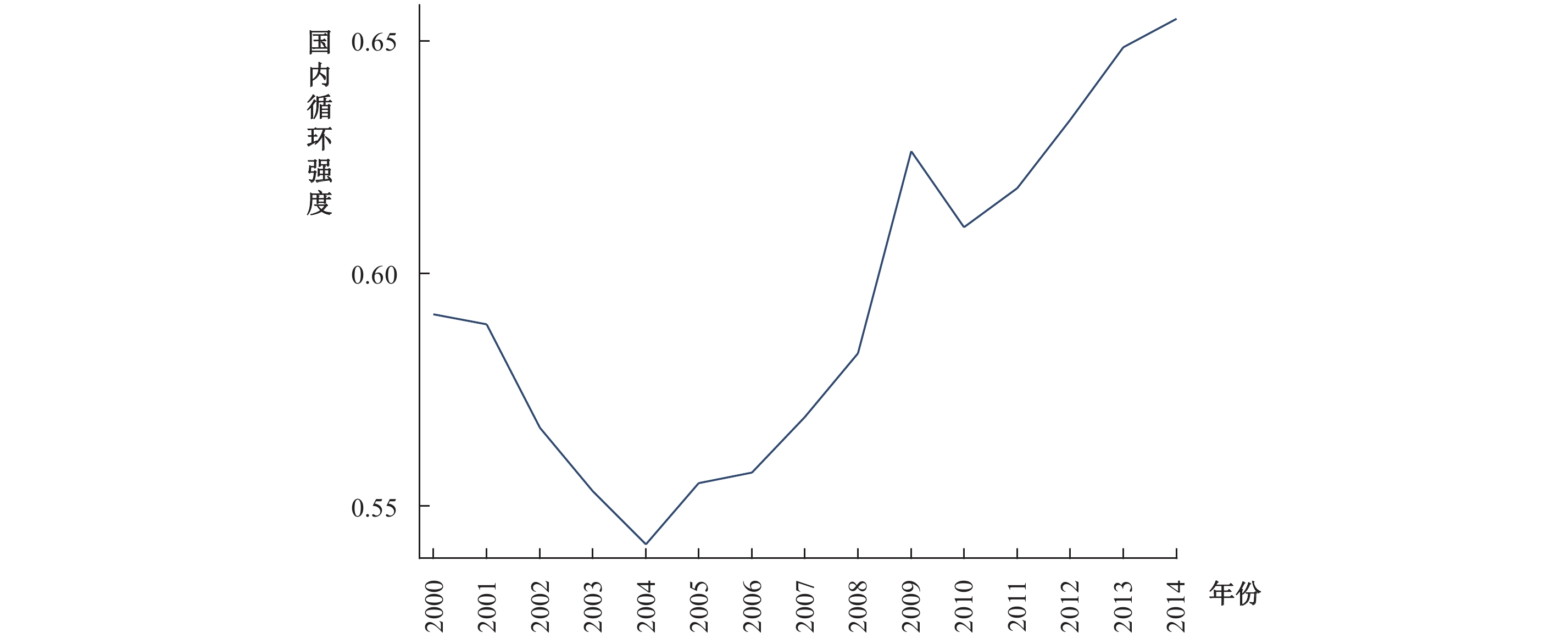图 2 中国制造部门的国内循环强度发展态势 注：作者根据WIOD数据核算而成。

 \begin{aligned} \Delta {\rm }IDC_t^R{\rm{ = }}& IDC_t^R{\rm{ - }}IDC_{t - 1}^R = IDS_t^R \times IDM_t^R - IDS_{t - 1}^R \times IDM_{t - 1}^R \\ = & \frac{1}{2}(IDS_t^R + IDS_{t - 1}^R) \times (IDM_t^R - IDM_{t - 1}^R) + \frac{1}{2}(IDM_t^R + IDM_{t - 1}^R) \times (IDS_t^R - IDS_{t - 1}^R) \\ = & \widetilde {IDS_t^R} \times \Delta IDM_t^R + \widetilde {IDM_t^R} \times \Delta IDS_t^R \end{aligned} (10)

$\widetilde {IDS_{it}^R} \times$
$\Delta IDM_{it}^R$
，二是国内市场效应
$\widetilde {IDM_{it}^R} \times \Delta IDS_{it}^R$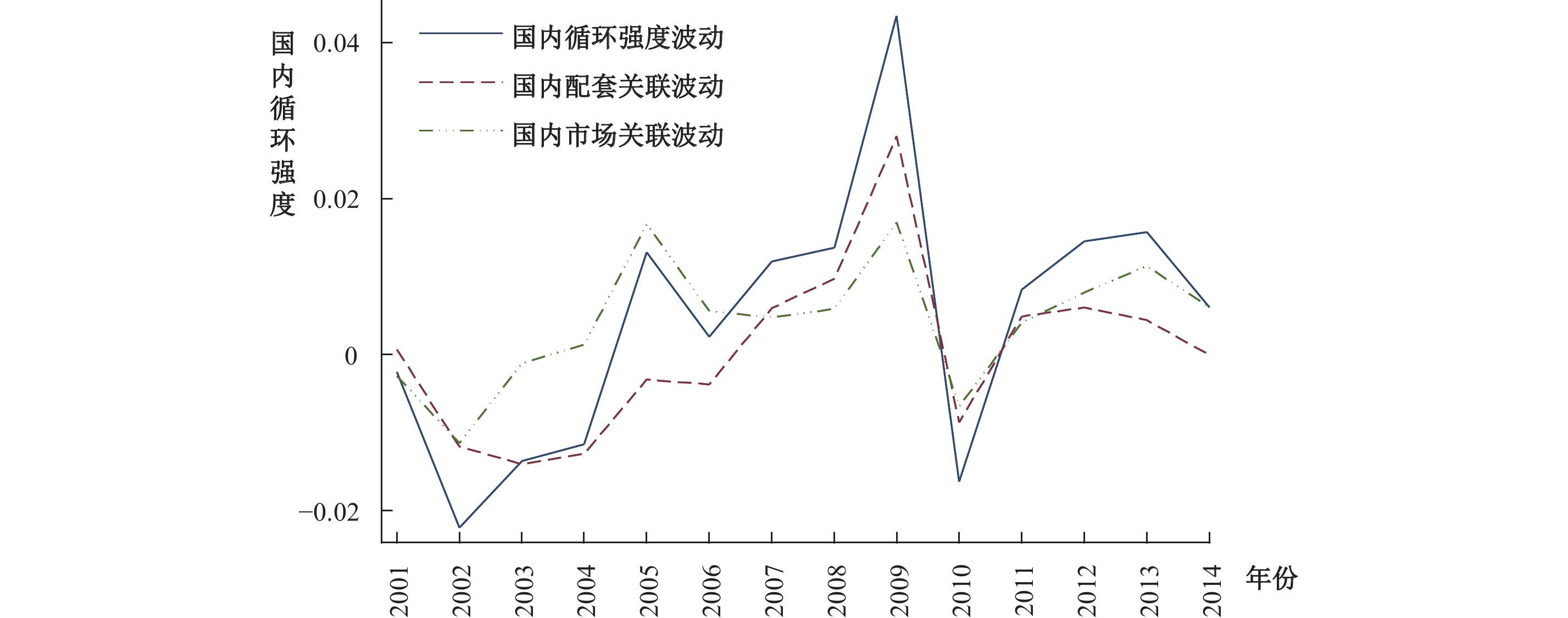图 3 中国制造部门国内循环强度波动的结构分解 注：作者根据WIOD数据核算而成。

2. 行业层面的核算。本文进一步对中国行业层面的国内循环强度进行统计核算。从横向来看，计算机及电子光学制造业、其他制造业这两个部门的国内循环强度较低，对进口中间品及国外市场的依赖程度相对较高。此外，中国大多数制造部门的国内循环强度均大于0.6，尤其以汽车制造、燃气电力的生产和供应、食品饮料烟草制造等行业最为显著，表明以上部门的上游配套基本布局在国内，其产品销售也更多地瞄准国内市场。图 4 中国典型制造部门的国内循环强度变化情况 注：作者根据WIOD数据核算而成。图 5 中国制造部门细分行业的国内循环结构特征（2014年） 注：（1）作者根据WIOD数据核算而成。（2）图中字母代表各工业部门，分别为：1（食品饮料烟草）、2（纺织鞋帽皮革）、3（木材、软木及制品）、4（造纸和纸制品）、5（印刷和记录媒介复制）、6（石油加工、炼焦及核燃料加工）、7（化学原料和化学制品）、8（医药制造）、9（橡胶塑料制品）、10（非金属矿物制品）、11（金属矿物制造）、12（金属制品）、13（计算机及电子光学）、14（电气设备制造）、15（机械设备制造）、16（汽车制造）、17（其他运输设备制造）、18（其他制造）、19（燃气电力的生产和供应）、20（水力的生产和供应）、21（废旧物资回收加工）。下同。

（二）中国参与国际循环的态势及特征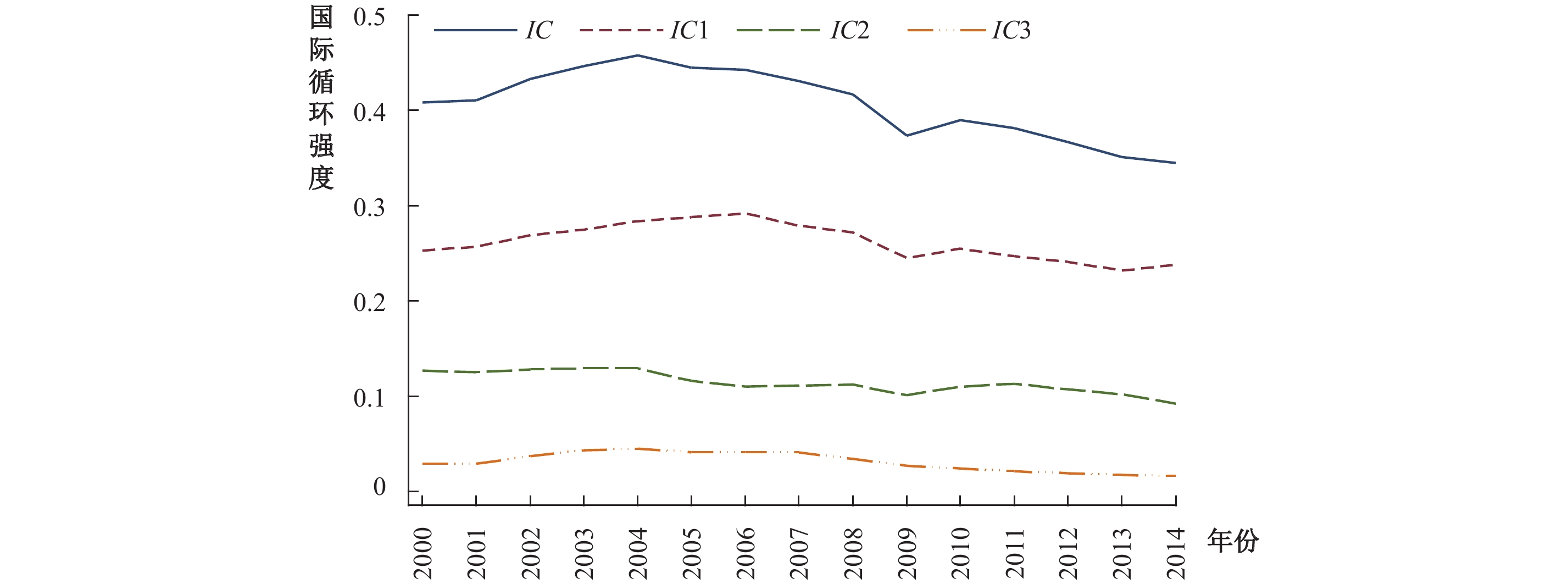图 6 2000年以来中国制造部门三种参与模式的国际循环强度波动态势 注：作者根据WIOD数据核算而成。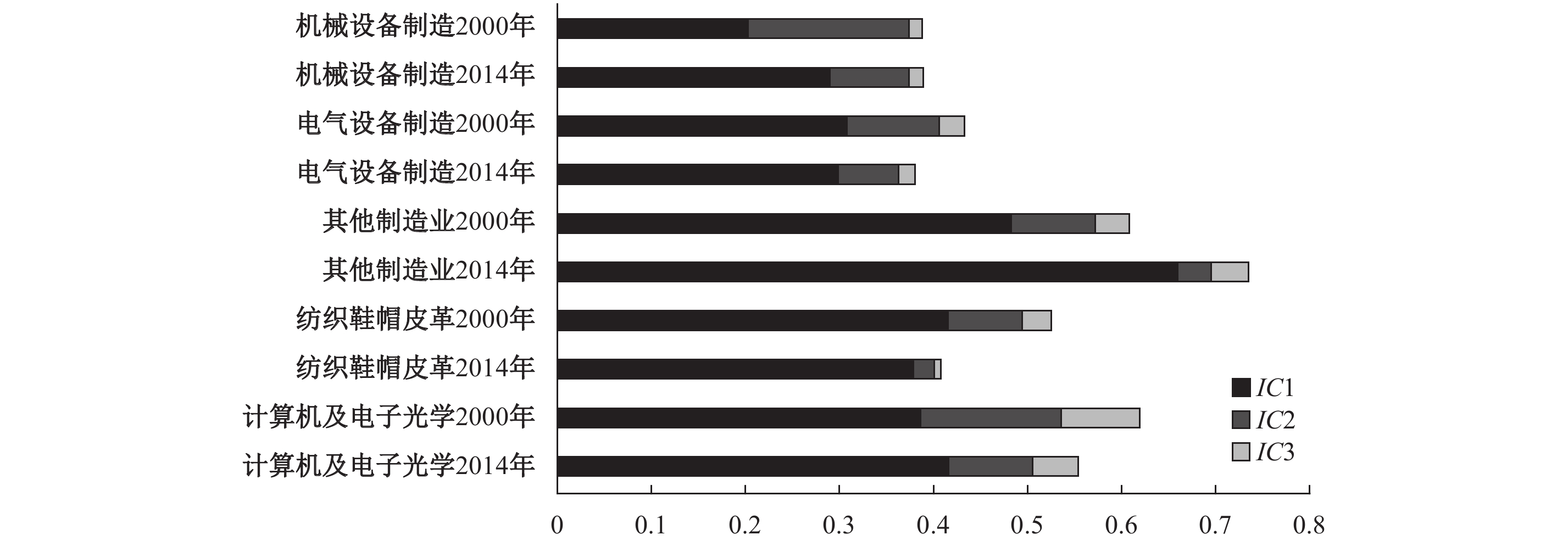图 7 中国主要制造部门三种参与模式的国际循环强度变化态势 注：作者根据WIOD数据核算而成。

（一）参与国际循环如何影响国内大循环图 8 外资进入与中国制造部门国内循环的关系（2014年） 注：（1）作者根据WIOD数据及《中国工业经济统计年鉴》核算而成。（2）图中数字代表各工业部门，含义同上。图 9 外资进入对典型制造部门国内市场关联的影响 注：作者根据WIOD数据及《中国工业经济统计年鉴》核算而成。

（二）国内大循环如何影响中国参与国际循环模式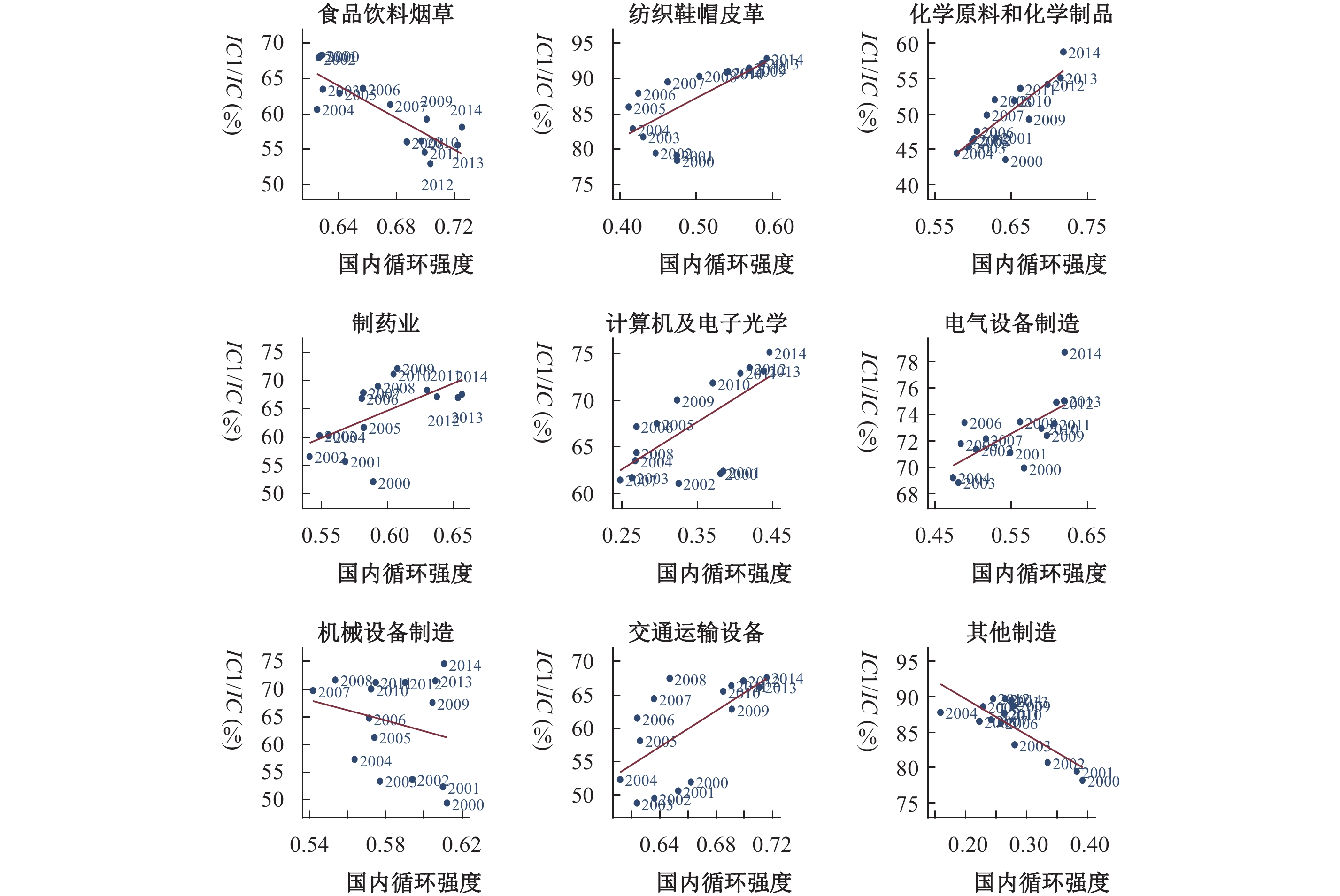图 10 中国主要制造部门国内循环对其国际循环参与模式的影响 注：作者根据WIOD数据核算而成。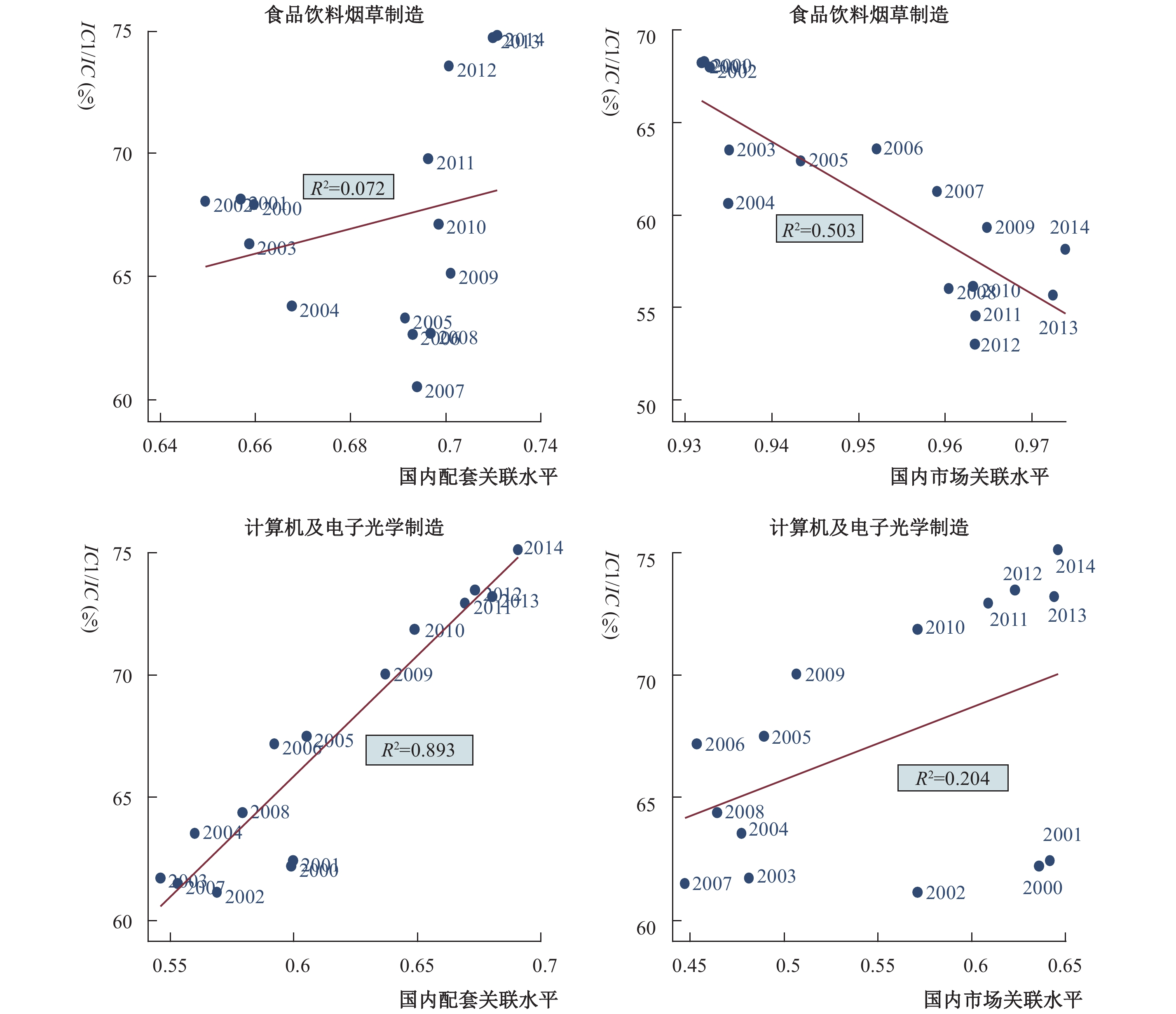图 11 中国典型制造部门不同国内循环形式对其国际循环参与模式的影响 注：作者根据WIOD数据核算而成。

① 因篇幅所限，相关图表未能在正文中呈现，读者可通过工作论文查询。

② 2014年汽车制造部门的产品内销占比高达95.45%。

③ 2014年计算机及电子光学制造部门的产品内销占比仅为45.86%。

④ 事实上，在一般贸易出口参与强度下降的同时，国内市场需求的扩大引致更大规模的一般贸易进口，食品饮料烟草制造部门的一般贸易进口模式参与国际循环强度占比迅速提升，该比重由2000年的1.72%增长为2014年的5.95%。

  包群, 叶宁华, 王艳灵. 外资竞争、产业关联与中国本土企业的市场存活[J]. 经济研究, 2015(7): 102–115.  崔向阳, 袁露梦, 钱书法. 区域经济发展: 全球价值链与国家价值链的不同效应[J]. 经济学家, 2018(1): 61–69.  杜宇玮. 产品差异化、GVC博弈与代工企业自主品牌升级[J]. 世界经济与政治论坛, 2020(4): 104–136. DOI:10.3969/j.issn.1007-1369.2020.04.006  黎峰. 增加值视角下的中国国家价值链分工——基于改进的区域投入产出模型[J]. 中国工业经济, 2016(3): 52–67.  黎峰. 进口贸易、本土关联与国内价值链重塑[J]. 中国工业经济, 2017a(9): 25–43.  黎峰. 外资进入如何影响了中国国内价值链分工?[J]. 财经研究, 2017b(11): 70–83.  黎峰, 曹晓蕾, 陈思萌. 中美贸易摩擦对中国制造供应链的影响及应对[J]. 经济学家, 2019(9): 104–112.  李跟强, 潘文卿. 国内价值链如何嵌入全球价值链: 增加值的视角[J]. 管理世界, 2016(7): 10–22.  刘志彪, 张杰. 全球代工体系下发展中国家俘获型网络的形成、突破与对策——基于GVC与NVC的比较视角[J]. 中国工业经济, 2007(5): 39–47.  刘志彪, 张杰. 从融入全球价值链到构建国家价值链: 中国产业升级的战略思考[J]. 学术月刊, 2009(9): 59–68.  刘志彪, 张少军. 中国地区差距及其纠偏: 全球价值链和国内价值链的视角[J]. 学术月刊, 2008(5): 49–55.  刘志彪. 重构国家价值链: 转变中国制造业发展方式的思考[J]. 世界经济与政治论坛, 2011(4): 1–14. DOI:10.3969/j.issn.1007-1369.2011.04.001  毛其淋, 盛斌. 贸易自由化、企业异质性与出口动态——来自中国微观企业数据的证据[J]. 管理世界, 2013(3): 48–68.  盛斌, 苏丹妮, 邵朝对. 全球价值链、国内价值链与经济增长: 替代还是互补[J]. 世界经济, 2020(4): 3–27.  张幼文. 要素流动下世界经济的机制变化与结构转型[J]. 学术月刊, 2020(5): 39–50.  Arndt S W. Globalization and the open economy[J]. The North American Journal of Economics and Finance, 1997, 8(1): 71–79. DOI:10.1016/S1062-9408(97)90020-6  Bazan L, Navas-Alemán L. The underground revolution in the Sinos Valley: A comparison of upgrading in global and national value chains[A]. Schmitz H. Local enterprises in the global economy: Issues of governance and upgrading[M]. Cheltenham: Edward Elgar Publishing Limited, 2004.  Beverelli C, Koopman R B, Kummritz V. Domestic value chains as stepping stones to global value chain integration[R]. WTO Working Paper No. 41, 2017.  Fally T. On the fragmentation of production in the US[R]. University of Colorado-Boulder Working Paper No.15, 2011.  Grossman G M, Helpman E. Integration versus outsourcing in industry equilibrium[R]. Cesifo Working Paper No.1, 2001.  Hummels D, Rapoport D, Yi K M. Vertical specialization and the changing nature of world trade[J]. Economic Policy Review, 1998, 4(2): 79–99.  Jones R W, Kierzkowski H. The role of services in production and international trade: A theoretical framework[R]. RCER Working Papers No.6, 1988.  Koopman R, Wang Z, Wei S J. Tracing value-added and double counting in gross exports[J]. American Economic Review, 2014, 104(2): 459–494. DOI:10.1257/aer.104.2.459  Meng B, Wang Z, Koopman R. How are global value chains fragmented and extended in China’s domestic production networks?[R]. IDE Discussion Papers No.424, 2013.  Raikes P, Jensen M F, Ponte S. Global commodity chain analysis and the French filière approach: Comparison and critique[J]. Economy and Society, 2000, 29(3): 390–417. DOI:10.1080/03085140050084589  Wang Z, Wei S J, Yu X D, et al. Characterizing global value chains: Production length and upstreamness[R]. NBER Working Papers No.23261, 2017.